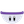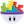#### Howdy, Stranger!

It looks like you're new here. If you want to get involved, click one of these buttons!

# sin formula, start position

Posts: 3Member, PROsin(|game.time|speed)distance+|start position|
How make RANDOM |start position| in this formula?
(+random(min, max)) does not work correctly

• Posts: 2,782Member, PROwhat do you mean by start position? The position on the scene or the starting angle?

• London, UK.Posts: 12,822Memberedited February 2018

(+random(min, max)) does not work correctly

It works correctly, it's probably just not doing what you want it to do.

. . . . . . . .

How make RANDOM start position in this formula?

If you are after a random starting angle (?) the formula would look like this . . .

sin((game.time`*`speed)+random(0,360))`*`distance+start position

If you are after a random start position the formula would look like this . . .

sin(game.time`*`speed)`*`distance+ random (A,B)

(use whatever values you like for A and B to set the range for the random value)